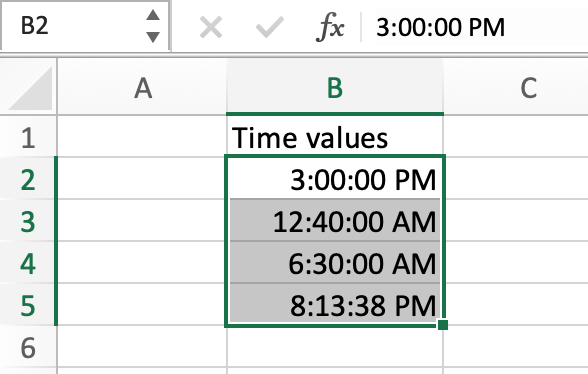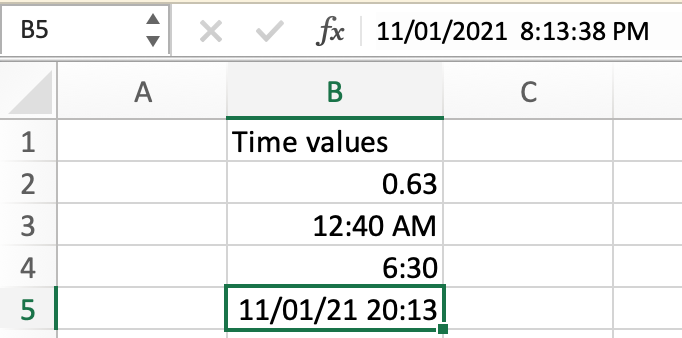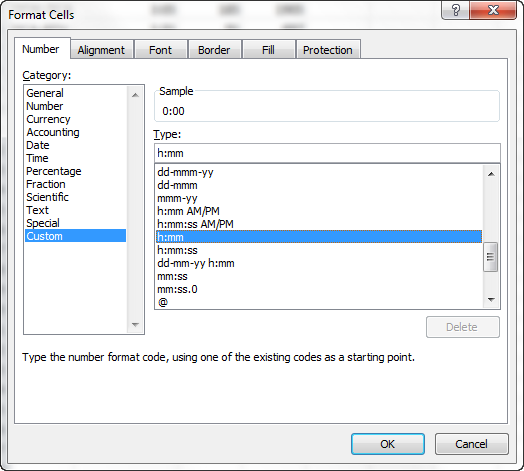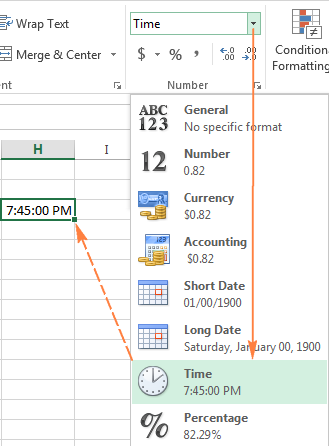# How to Enter Time values Quickly in ExcelIn this article, we will learn How to Enter Time values Quickly in Excel

Scenario:

Excel considers only two forms of cell value: text and number. Numbers include many formats like General, Scientific, date, time, currency, fraction, percentage and accounting. These all formats have different custom changes and can be converted to each other. Change number formats from custom formats option

Time formats in Excel

To open Format cell option. First select the cell or range of cells and use the shortcut key Ctrl + 1.

There are many time formats in the Format cells option. Some of these are:

• h:mm AM/PM
• h:mm:ss AM/PM
• h:mm
• h:mm:ss
• dd/mm/yy hh:mm AM/PM
• dd/mm/yy hh:mm:ss AM/PM

The last two can be used with both date and time formats.

Example :

All of these might be confusing to understand. Let's understand how to use the function using an example. Here we have some time values in different formats.And we need to change their formats to one specific time format hh:mm:ss.

For this First select the cells where date formatting is required. Now Use Ctrl + 1 to open Format cells dialog box.Select Custom or time category. You can either type the specific format or choose from the given ones. Select the one and Click Ok.As you can see you get all the time values under one format. Or you can select the cells and right click > Format cells option to open Format cell dialog box.

Alternate way

This process is common practice to change cell format. Go to Home > Change General to Time (under Number).As you can see from the picture above.

Here are all the observational notes using the formula in Excel
Notes :

1. Excel stores dates and time as numbers and is used in calculation by the function. So it’s recommended to use dates as cell reference or using the TIME function instead of giving direct argument to the function. Check the validation of time values, or else the function returns #VALUE! Error.
2. Excel TIMEVALUE function only converts time values in text format to time values in number format.

Hope this article about How to Enter Times Quickly in Excel is explanatory. Find more articles on calculating values and related Excel formulas here. If you liked our blogs, share it with your friends on Facebook. And also you can follow us on Twitter and Facebook. We would love to hear from you, do let us know how we can improve, complement or innovate our work and make it better for you. Write to us at info@exceltip.com.

Related Articles :

Excel converts decimal Seconds into time format : As we know that time in excel is treated as numbers. Hours, Minutes, and Seconds are treated as decimal numbers. So when we have seconds as numbers, how do we convert into time format? This article got it covered.

Count holidays between dates in Excel : count non working days between the two given dates using the DATEDIF and NETWORKDAYS function in Excel.

Extract days between dates ignoring years in Excel : count the days between the two dates using different functions and mathematical operation in Excel

Count Birth Dates By Month in Excel : count the number of dates lying in a particular month using the SUMPRODUCT and MONTH function in Excel.

SUM price by weekdays in Excel : sum price values corresponding to the number of dates in a particular weekday using the SUMPRODUCT and WEEKDAY function in Excel.

How to Convert date to text in Excel : In this article we learned how to convert text into date, but how do you convert an excel date into text. To convert an excel date into text we have a few techniques.

How to use the NETWORKDAYS function in Excel : Returns the working days between the two given dates using the NETWORKDAYS function.

Popular Articles :

How to use the IF Function in Excel : The IF statement in Excel checks the condition and returns a specific value if the condition is TRUE or returns another specific value if FALSE.

How to use the VLOOKUP Function in Excel : This is one of the most used and popular functions of excel that is used to lookup value from different ranges and sheets.

How to use the SUMIF Function in Excel : This is another dashboard essential function. This helps you sum up values on specific conditions.

How to use the COUNTIF Function in Excel : Count values with conditions using this amazing function. You don't need to filter your data to count specific values. Countif function is essential to prepare your dashboard.

Terms and Conditions of use

The applications/code on this site are distributed as is and without warranties or liability. In no event shall the owner of the copyrights, or the authors of the applications/code be liable for any loss of profit, any problems or any damage resulting from the use or evaluation of the applications/code.